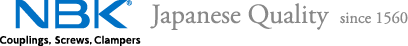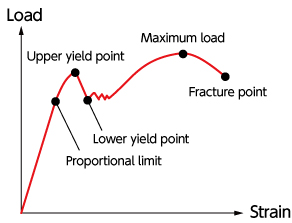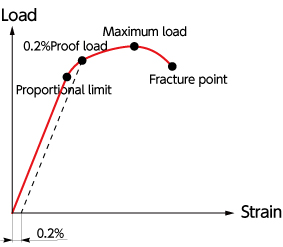#Date Published:Sep. 27. 2016

# Vol.2　Explaining the Vocabulary of ScrewsHello, Nedzigon here!
Today we are going to be talking about terms you should know when dealing with screws.
You may already know a lot of them, but stay with me for a review.

#### Tightening Torque

Tightening torque is the force required to rotate a screw. The relation between tightening torque and axial force in the elastic region is expressed by the following formula:

T=kdF
T：Tightening torque (N·m)
k：Torque coefficient
d：Screw nominal diameter
F：Axial force (N)The torque method refers to managing tightening torque with a torque wrench etc. in order to control the axial force on the screw. Because it can be done with a tool and is a relatively simple way of managing axial force, it is widely used; however, as will be described later, the torque coefficient varies: this means that axial force varies more than with other methods, requiring attention.

#### Torque Coefficient

Torque coefficient is the value determined by the friction coefficient of the screw portion and the seating surface. It differs depending on the material, the surface roughness, the use of plating or oil and so on, but is generally said to be about 0.15 to 0.25.

#### Axial Force

Axial force is the force (tightening force) generated in the axial direction when the screw is tightened.

Yield load is the load at which the material deforms beyond return to its original state. When load and stretch are increased linearly in tension testing, the load suddenly decreases and the stretch alone continues to increase. This is known as the yield phenomenon, while the load during this time is known as the yield load.
The value of yield load divided by cross-sectional area is the yield stress.#### 0.2% Proof Load / Plastic Strain

0.2% Proof Load is the load that generates 0.2% plastic strain when external force is removed from metal material such as aluminum alloy which does not demonstrate the yield phenomenon. It is used in place of yield load.
Plastic strain that persists even after the external force is removed is known as permanent strain. Conversely, elastic strain is strain that decreases to 0 when external force is removed.#### Tensile Strength

Tensile strength is an index for strength levels, referring to the maximum tensile stress the material can endure.
Given material variance, the value below which the material will not break is called the minimum tensile strength.

#### Strength Class

Strength class is an index for screw strength, displayed differently for steel screws and stainless steel screws.
Carbon steel and alloy steel screws are displayed as "10.9" or "12.9", with the ones place showing the 1/100 value of nominal tensile strength and the tenths place showing a value 10 times the ratio of nominal lower yield point and nominal tensile strength. For example, "12.9" shows a nominal tensile strength of 1200 N/mm2 with nominal proof stress of 1200 x 0.9 for 1080 N/mm2.
"A2-70" is displayed for stainless steel screws, with the numbers before the hyphen representing the type of steel, and the numbers after it the strength class, indicating 1/10 the tensile strength value. For example, "A2-70" has a minimum tensile strength of 700 N/mm2.

There are many other terms that one should know in addition to these when dealing with screws, but that's for another time.

That's it for today.